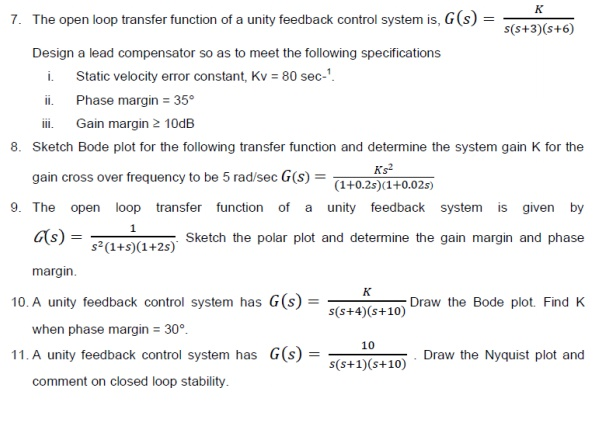Home | | Control System Engineering | Important Short Questions, Answers, Tutorial Problems: Frequency Response Analysis

# Important Short Questions, Answers, Tutorial Problems: Frequency Response Analysis

Control Systems - Frequency Response Analysis - Important Short Questions, Answers, Tutorial Problems: Frequency Response Analysis

FREQUENCY RESPONSE

1.           What is frequency response?

A frequency response is the steady state response of a system when the input to the system is a sinusoidal signal.

2.           List out the different frequency domain specifications?

The frequency domain specifications are

Resonant peak.

Resonant frequency.

Bandwidth

Cut-off rate

Gain margin

Phase margin

3.           Define –resonant Peak

The maximum value of the magnitude of closed loop transfer function is called resonant peak.

4.           What is bandwidth?

The bandwidth is the range of frequencies for which the system gain Is more than 3 dB. The bandwidth is a measure of the ability of a feedback system to reproduce the input signal ,noise rejection characteristics and rise time.

5.           Define Cut-off rate?

The slope of the log-magnitude curve near the cut-off is called cut-off rate. The cut-off rate indicates the ability to distinguish the signal from noise.

6.           Define –Gain Margin?

The gain margin, kg is defined as the reciprocal of the magnitude of the open loop transfer function at phase cross over G(jω frequency. Gain margin kg

7.           Define Phase cross over?

The frequency at which, the phase of open loop transfer functions is 180° is called phase cross over frequency ωpc.

8. What is phase margin?

It is the amount of phase lag at the gain cross The phase margin ,γ over frequency required to bring system to the verge of instability.

9. Define Gain cross over?

The gain cross over frequency ωgc is the frequency at which the magnitude of the open loop transfer function is unity..

10. What is Bode plot?

The Bode plot is the frequency response plot of the transfer function of a system. A Bode plot consists of two graphs. One is the plot of magnitude of sinusoidal transfer function versus log ω.The other is a plot of the phase angle of a sinusoidal function versus log ω.

11. What are the main advantages of Bode plot?

i) Multiplication of magnitude can be in to addition.

ii) A simple method for sketching an approximate log curve is available.

iii) It is based on asymptotic approximation. Such approximation is sufficient if rough information on the frequency response characteristic is needed. is available.

iv) The phase angle curves can be easily drawn if a template for the phase angle curve of 1+ jω

12. Define Corner frequency?

The frequency at which the two asymptotic meet in a magnitude plot is called corner frequency.

13. Define Phase lag and phase lead?

A negative phase angle is called phase lag. A positive phase angle is called phase lead.

14. What are M circles?

The magnitude M of closed loop transfer function with unity feedback will be in the form of circle in complex plane for each constant value of M. The family of these circles are called M circles.

15. What is Nichols chart?

The chart consisting if M & N loci in the log magnitude versus phase diagram is called Nichols chart.

16. What are two contours of Nichols chart?

Nichols chart of M and N contours, superimposed on ordinary graph. The M contours are the magnitude of closed loop system in decibels and the N contours are the phase angle locus of closed loop system.

17. What is non-minimum phase transfer function?

A transfer function which has one or more zeros in the right half S-plane is known as non-minimal phase transfer function.

18. What are the advantages of Nichols chart?

i) It is used to find the closed loop frequency response from open loop frequency response.

ii)  Frequency domain specifications can be determined from Nichols chart.

iii) The gain of the system can be adjusted to satisfy the given specification.

19. What are N circles?

If the phase of closed loop transfer function with unity feedback is α, then N=tan α. For each constant value of N, a circle can be drawn in the complex plane. The family of these circles are called N circles.

20. What are the two types of compensation?

The two types of compensation are

ii. Feedback compensation or parallel compensation.

21. What are the three types of compensators?

The three types of compensators are

i. Lag compensator.

22. What are the uses of lead compensator?

The uses of lead compensator are

speeds up the transient response

increases the margin of stability of a system

increases the system error constant to a limited extent.

23. What is the use of lag compensator?

The lag compensator Improve the steady state behavior of a system, while nearly preserving its transient response.

24. When lag-lead compensator is is required?

The lag lead compensator is required when both the transient and steady state response of a system has to be improved

25. What is a compensator?

A device inserted into the system for the purpose of satisfying the specifications is called as a compensator.

Lag compensation is employed for a stable system for improvement in steady state performance. Lead compensation is employed for stable/unstable system for improvement in transient state performance.

Lag-Lead compensation is employed for stable/unstable system for improvement in both steady state and transient state performance

27. What are the effects of adding a zero to a system?

Adding a zero to a system results in pronounced early peak to system response thereby the peak overshoot increases appreciably.Study Material, Lecturing Notes, Assignment, Reference, Wiki description explanation, brief detail
Control Systems : Frequency Response Analysis : Important Short Questions, Answers, Tutorial Problems: Frequency Response Analysis |